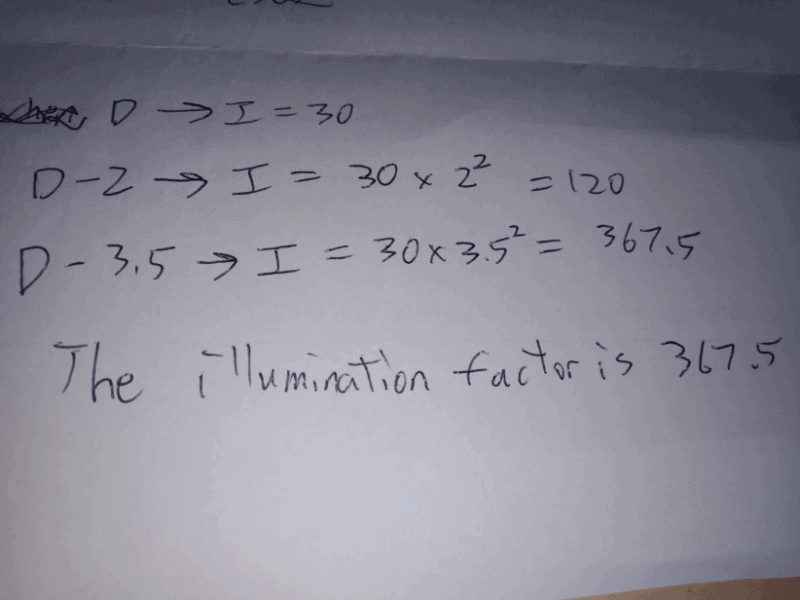# Question

Equation 1, D , I = 30

I = k/D^2

30 = k/D^2

k = 30 D^2  ——- (1)

Equation 2, D-2, I = 120

I = k/(D-2)^2

120 =  k/(D-2)^2  —— (2)

Sub (1) to (2), you will get

3D^2 -16D +16 = 0

Find D, D = 4

sub D into (1) and (2),

both equation you will get k = 480

Answering the question, sub D=4 and k= 480

I  = k/(D-3.5)^2

I =480/0.5^2

I = 1920

0 Replies 1 Like ✔Accepted Answer